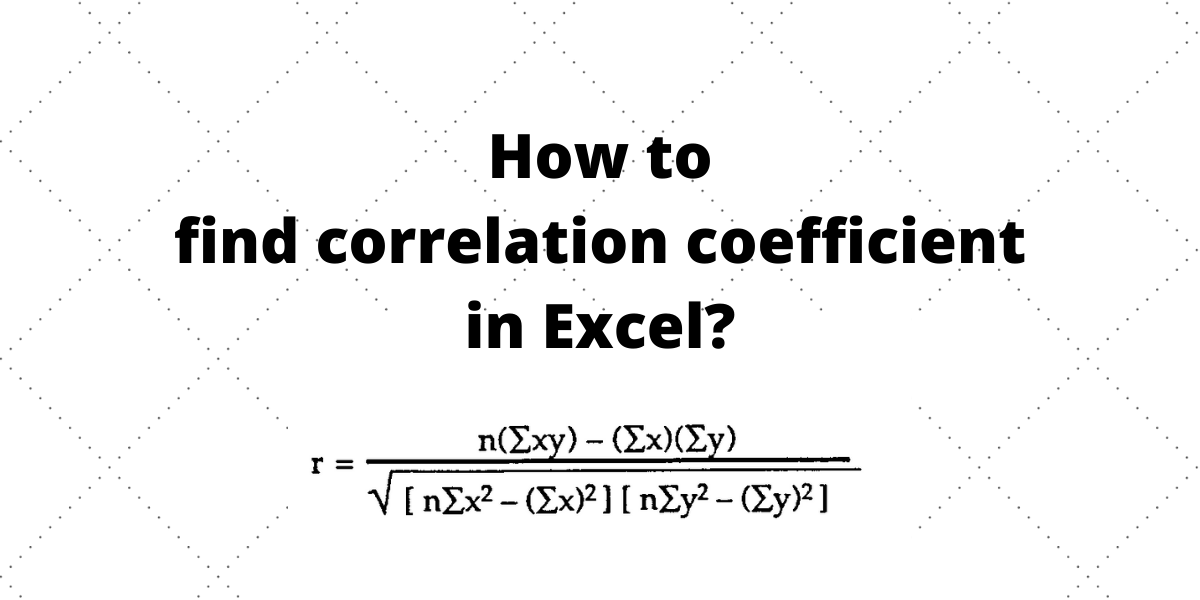# How to find the correlation coefficient in Excel?The correlation coefficient is a measurement of how strongly two variables are related to each other. In Excel, we have the inbuilt CORREL function to find the correlation coefficient between two variables.

Also read: Calculate the Square Root in Excel

## What is the Pearson Correlation Coefficient?

The Pearson Correlation Coefficient is another name for the correlation coefficient, which indicates how two variables are related. The correlation coefficient has a value between -1 and +1. A correlation value of 0 (zero) shows that there is no correlation.

A perfect positive correlation is represented by a correlation coefficient of +1, which signifies that as the independent variable rises, the other variable rises, and as the independent variable falls, the other variable falls. The higher the correlation, the closer the coefficient is to +1.

A perfect negative correlation has a correlation value of -1, which means that as the independent variable rises, the other variable falls, and as the independent variable falls, the other variable rises. The weaker the correlation, the closer the coefficient is to -1.

## Using the CORREL function

The CORREL function returns the coefficient of correlation between two cell ranges.

Syntax: `CORREL(array1,array2)`, where array1 is a range of cell values and array2 is the second range of cell values.

Let us consider a dataset of height (in) and weight (lbs) for a sample of people. We want to know if these two variables are related.

• Here, array1 (range of height values) is A2:A11 and array2 (range of weight values) is B2:B11. The formula for correlation calculation is =CORREL(A2:A11,B2:B11)

A correlation coefficient of 0.847299365 indicates a high correlation between height and weight for the sample of people.

Note:

• The CORREL function produces a #N/A error if array1 and array2 contain different numbers of data points.
• The CORREL method produces a #DIV/0! error if either array1 or array2 is empty.

## Conclusion

This article helps you to calculate correlation coefficient with ease using a function in Excel rather than by formula which is a tedious process.